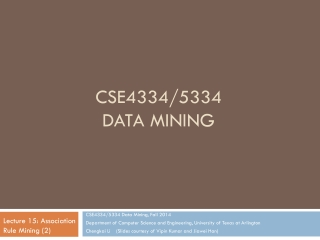DownloadDownload PresentationCSE4334/5334 Data Mining

# CSE4334/5334 Data Mining

Download Presentation## CSE4334/5334 Data Mining

- - - - - - - - - - - - - - - - - - - - - - - - - - - E N D - - - - - - - - - - - - - - - - - - - - - - - - - - -
##### Presentation Transcript

1. CSE4334/5334 Data Mining CSE4334/5334 Data Mining, Fall 2014 Department of Computer Science and Engineering, University of Texas at Arlington Chengkai Li (Slides courtesy of Vipin Kumar and Jiawei Han) Lecture 15: Association Rule Mining (2)

2. Pattern Evaluation

3. Pattern Evaluation • Association rule algorithms tend to produce too many rules • many of them are uninteresting or redundant • Redundant if {A,B,C}  {D} and {A,B}  {D} have same support & confidence • Interestingness measures can be used to prune/rank the derived patterns • In the original formulation of association rules, support & confidence are the only measures used

4. There are lots of measures proposed in the literature Some measures are good for certain applications, but not for others What criteria should we use to determine whether a measure is good or bad? What about Apriori-style support based pruning? How does it affect these measures?

5. f11: count of X and Yf10: count of X and Yf01: count of X and Yf00: count of X and Y Computing Interestingness Measure • Given a rule X  Y, information needed to compute rule interestingness can be obtained from a contingency table Contingency table for X  Y Used to define various measures • support, confidence, lift, Gini, J-measure, etc.

6. Association Rule: Tea  Coffee Confidence= P(Coffee|Tea) = 0.75 but P(Coffee) = 0.9 • Although confidence is high, rule is misleading • P(Coffee|Tea) = 0.9375 Drawback of Confidence

7. Statistical Independence • Population of 1000 students • 600 students know how to swim (S) • 700 students know how to bike (B) • 420 students know how to swim and bike (S,B) • P(SB) = 420/1000 = 0.42 • P(S)  P(B) = 0.6  0.7 = 0.42 • P(SB) = P(S)  P(B) => Statistical independence • P(SB) > P(S)  P(B) => Positively correlated • P(SB) < P(S)  P(B) => Negatively correlated

8. Statistical-based Measures • Measures that take into account statistical dependence = 1, independent > 1, positively correlated < 1, negatively correlated = 0, independent > 0, positively correlated < 0, negatively correlated

9. Example: Lift/Interest Association Rule: Tea  Coffee Confidence= P(Coffee|Tea) = 0.75 but P(Coffee) = 0.9 • Lift = 0.75/0.9= 0.8333 (< 1, therefore is negatively associated)

10. Drawback of Lift & Interest Statistical independence: If P(X,Y)=P(X)P(Y) => Lift = 1

11. Example: -Coefficient Association Rule: Tea  Coffee (< 0, therefore is negatively correlated)

12. Drawback of -Coefficient  Coefficient is the same for both tables